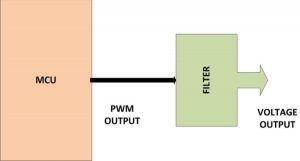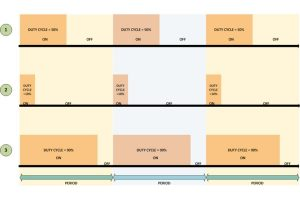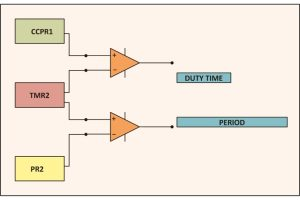# How to Glow an LED using PWM with PIC Microcontroller- (Part 20/25)

Pulse Width Modulation (PWM) is a technique in which the width of a pulse is modulated keeping the time period of the wave constant. One cycle has a fixed time period called ‘Period’ and a varying on time called ‘Duty cycle’. The entire wave can have two voltages levels either logic 0 or logic 1. The PWM wave is very useful in the digital systems, since this can be used to generate different voltage values other than the logic 0 or logic 1 values. This feature is making use in so many digital systems like DC motor control, audio devices, simple decoration light controls etc.

The PIC18F4550 has an inbuilt PWM module which can generate continuous PWM waves. The Period and the duty cycle of the PWMwave can be adjusted in program. This project explores the PWM module of the PIC18F4550 and tries to glow an LED with varying intensities, which shows that it is possible to generate any required voltage at a pin of a digital microcontroller with the help of PWM waves.

PWM is a digital wave that can be generated using digital circuits which are not capable of generating analog voltages. With the help of the modulation of the width of a pulse in a period of the wave, they can generate any required voltage with the help of a proper filter circuits. Thus the PWM wave is always associated with a filter circuit which has been designed according to the timing details of the pulse.

The following figure shows a microcontroller generating PWM wave which is then used to generate the corresponding analog voltage with the help of a filter circuit.The filter could be any device which can generate the equivalent voltage of a PWM voltage. It normally consists of Low-pass filters, amplifiers, load drivers etc. They generate the voltage and fed that voltage to the load device. In certain cases where the load itself can act as a filter generating the voltage from the PWM wave and drive itself using that voltage. The example of such a devices which can itself act as a PWM filter is DC motor. In this particular project also there is no filter circuit since the aim is to vary the intensity of a glowing LED only. It doesn’t mean that the LED can act as a filter; rather it is the persistence of vision that makes one feel that the intensity of the LED is varying. In this case the LED is glowing normally for the logic1 and logic0 voltages of a PWM, but the variation in the ON time make one feel that the intensity is varying. Here the persistence of vision plays the key role and the filter device is nothing but our eyes itself.

The PIC18F4550 has four PWM output channels and they are P1A, P1B, P1C and P1D. All of them are capable of generating PWM waves at a time. In this project only one of the PWM channels are using. The P1A is the PWM channel in this particular project. A simple example of the of waves generated at this pin is shown below;As shown in the above figure the ‘Period’ of all the wave remains the same but their ‘Duty-cycle’ varies.

The period of the wave is the sum of the ‘ON time + OFF time’. Duty-cycle is the percentage of time period for which the logic1 voltage exists in a cycle (ON time), starting from the beginning of the cycle.

The PWM is that kind of a wave in which the ON time and OFF time can vary in a cycle but the sum of ‘ON time + OFF time’ remains constant for every cycle.

The period and the duty cycle for a PWM wave can be calculated generally using the following equations;

Period = ON time + OFF time

Duty-cycle = ON time / (ON time + OFF time) = ON time / Period

As shown in the figure, the first PWM wave has a Duty-cycle of 50% which means the on-time is exactly half of that the period of the wave. The second wave has 10% Duty-cycleand the third wave has 90% Duty-cycle. When applied to a proper filter the 50% duty cycle can produce half of the maximum voltage of the pulse. If the maximum voltage (logic 1 voltage) of the pulse is 5V then the 50% duty cycle wave can produce a continuous 2.5V. The 10% duty cycle wave can produce nearly 0 voltages and the 90% duty cycle wave can produce nearly 5V.Thus the wave when applied to a filter can continuously produce a voltage which is the average of the voltage in a single period.

Increasing the Duty-cycle will increase the voltage at the filter device’s output and decreasing the Duty-cycle will decrease the voltage as well

The filter could be anything like a capacitor or inductor. Even though if there is no filter most of the device can generate a corresponding output with a PWM wave resembles that the device is operating at the average voltage of that PWM wave. The LED in this project is the simplest example for such a kind of device.

The registers:

The registers which help in generating the Period and the Duty cycle of the PWM module in a PIC18F4550 are CPR1, PR2 and TMR2. The TMR2 and the PR2 are the timer value and the Pre-scalar value registers of the Timer2 module. They are used to generate the Period of the PWM wave. The CCPR1 is a 10 bit register which can be used to set the duty cycle of the PWM wave.

Suppose one have written the required values into the PR2 and the CCPR1 registers for the Period and Duty cycle of the PWM wave. The TMR2 value is always set to zero once the PWM module is enabled. Once the module is enabled then with each clock the timer2 receives, the value in the TMR2 increases. Hence these registers act like up-counters. This value in the TMR2 register is always under a comparison with the values in the PR2 register and the CCPR1 register like as shown in the following figure;As per the above figure, the Duty-cycle (on-time) is available until the TMR2 value exceeds the CCPR1 value, since the Duty-cycle is the output of the comparator which compares the TMR2 value with the CCPR1 value. The Period of the wave is available further until the value in the TMR2 exceeds the PR2 value since the Period is the output of the comparator which compares the TMR2 value with the PR2 value

Once the value in the TMR2 exceeds that of the PR2 then the TMR2 is cleared to zero and starts counting again. Hence continuous PWM wave with specified duty cycle and Period is available at the PWM output pin. Once can rewrite the CCPR1 value any time to change the duty cycle of the PWM wave so as to change the voltage which the wave is generating at the filter device connected to the PWM pin of the microcontroller.

The Period of the PWM wave in PIC18F4550 given by;

PWM Period = [(PR2) + 1] * 4 * TOSC * (TMR2 Pre-scale Value)

The Duty cycle of the PWM wave in PIC18F4550 is given by;

PWM Duty Cycle = (CCPR1 * TOSC * (TMR2 Pre-scale Value)

CCP1CON

This is the register which controls the PWM operation in PIC18F4550. It can be used to configure the PWM output channels and enable the PWM module in the required mode and can also be used to set the lower two bits of the duty cycle value.

#### Current Project / Post can also be found using:

• microchip microcontroller projects
• microprocessor smd led projects
• micro controller with pwm output